Which is the most suitable reagent among the following to distinguish compound (III) from rest of the compounds?

I. CH3-C$\equiv$C-CH3

II. CH3-CH2-CH2-CH3

III. CH3-CH2-C$\equiv$CH

IV. CH3-CH=CH2

(a) Br2/CCl4                     (b) Br2/CH3COOH

(c)Alk.KMnO4                   (d) Ammonical AgNO3

Complete Question Bank + Test Series
Complete Question Bank

Difficulty Level:

Which of the following reagents will be able to distinguish between 1-butyne and 2-butyne?

(a) NaNH2            (b) HCl             (c) O2                 (d) Br2

Complete Question Bank + Test Series
Complete Question Bank

Difficulty Level:

A compound is treated with NaNH2 to give sodium salt. Identify the compound.

(a) C2H2                  (b) C6H6

(c) C2H6                   (d) C2H4

Complete Question Bank + Test Series
Complete Question Bank

Difficulty Level:

The product of the reaction between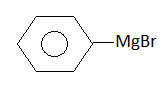+$\left({\mathrm{CH}}_{3}{\right)}_{3}\mathrm{COH}\to$ ?

(1)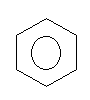(2)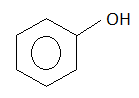(3)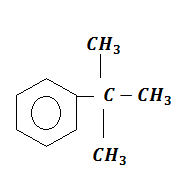(4)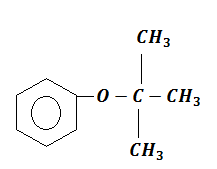Complete Question Bank + Test Series
Complete Question Bank

Difficulty Level:

The reaction of toluene with Cl2 in the presence of FeCl3 gives 'X' and reaction in presence of presence of light gives 'Y'. Thus 'X' and 'Y' are

(a) X= benzal chloride, Y=o-chlorotoluene

(b) X=m-chlorotoluene, Y=p-chlorotoluene

(c) X=o and p-chlorotoluene, Y= trichloromethyl benzene

(d) X= benzyl hloride, Y=m-chlorotoluene

Complete Question Bank + Test Series
Complete Question Bank

Difficulty Level:

What products are formed when the following compound is treated with Br2 in the presence of FeBr3?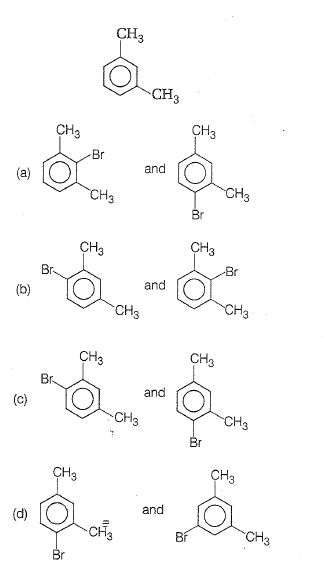Complete Question Bank + Test Series
Complete Question Bank

Difficulty Level:

When chlorine is passed through propene at 400$°$C, which of the following is formed?

(a) PVC                         (b) Allyl chloride

(c) Nickel chloride          (d) 1,2-dichloro ethane

Complete Question Bank + Test Series
Complete Question Bank

Difficulty Level:

In Friedel-Craft's alkylation, besides AlCl3 the other reactants are

(a) C6H6 + NH2

(b) C6H6 + CH4

(c) C6H6 + CH3Cl

(d) C6H6 + CH3COCl

Complete Question Bank + Test Series
Complete Question Bank

Difficulty Level:

Benzene reacts with n-propyl chloride in the presence of anhydrous AlCl3 to give

(a) 3-propyl-1-chlorobenzene

(b) n-propyl benzene

(c) no reaction

(d) iso-propyl benzene

Complete Question Bank + Test Series
Complete Question Bank

Difficulty Level:

When acetylene is passed through $\mathrm{dil}.{\mathrm{H}}_{2}{\mathrm{SO}}_{4}$ in presence of ${\mathrm{HgSO}}_{4}$, the compound formed is

(a) ether                           (b) ketone

(c) acetic acid                    (d) acetaldehyde